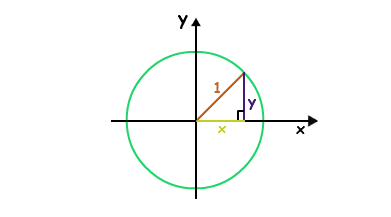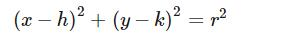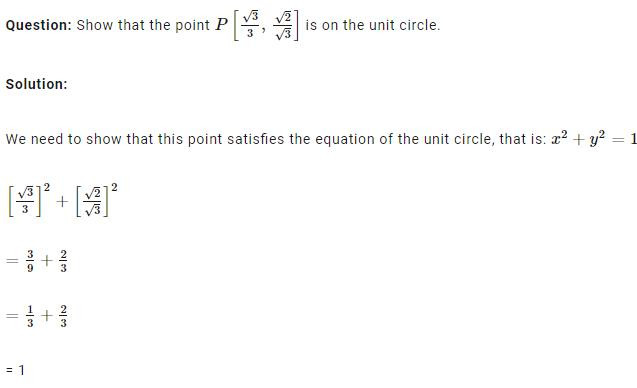Courses

# UNIT CIRCLE - Class 11 Class 11 Notes | EduRev

## Class 11 : UNIT CIRCLE - Class 11 Class 11 Notes | EduRev

The document UNIT CIRCLE - Class 11 Class 11 Notes | EduRev is a part of Class 11 category.
All you need of Class 11 at this link: Class 11

what is unit circle

Ref: https://edurev.in/question/715848/what-is-unit-circle-Related-Circle-and-Its-Equatio

UNIT CIRCLE FORMULA

In mathematics, a unit circle is a circle with a radius of one. Especially in trigonometry, the unit circle is the circle of radius one centered at the origin (0, 0) in the Cartesian coordinate system in the Euclidean plane.

The unit circle is often denoted S1 : the generalization to higher dimensions is the unit sphere. The interior of the unit circle is called the open unit disk while the interior of the unit circle combined with the unit circle itself is called the closed unit disk.The general equation of circle is given below:Where (h, k) are center coordinates and r is the radius.

The unit circle formula is:
x^2 + y^2

Where x and y are the coordinate values.

### SOLVED EXAMPLES UNIT CIRCLE FORMULATherefore P is on the unit circle.

Offer running on EduRev: Apply code STAYHOME200 to get INR 200 off on our premium plan EduRev Infinity!

,

,

,

,

,

,

,

,

,

,

,

,

,

,

,

,

,

,

,

,

,

;